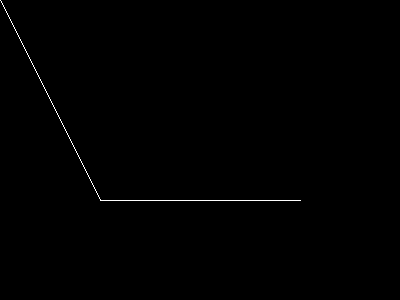# imageopenpolygon

(PHP 7 >= 7.2.0)

imageopenpolygonDraws an open polygon

### Description

bool imageopenpolygon ( resource `\$image` , array `\$points` , int `\$num_points` , int `\$color` )

imageopenpolygon() draws an open polygon on the given `image`. Contrary to imagepolygon(), no line is drawn between the last and the first point.

### Parameters

``` image```

An image resource, returned by one of the image creation functions, such as imagecreatetruecolor().

`points`

An array containing the polygon's vertices, e.g.:

 points = x0 points = y0 points = x1 points = y1

`num_points`

Total number of points (vertices).

`color`

A color identifier created with imagecolorallocate().

### Return Values

Returns `TRUE` on success or `FALSE` on failure.

### Examples

Example #1 imageopenpolygon() example

``` <?php// Create a blank image\$image = imagecreatetruecolor(400, 300);// Allocate a color for the polygon\$col_poly = imagecolorallocate(\$image, 255, 255, 255);// Draw the polygonimageopenpolygon(\$image, array(        0,   0,        100, 200,        300, 200    ),    3,    \$col_poly);// Output the picture to the browserheader('Content-type: image/png');imagepng(\$image);imagedestroy(\$image);?> ```

The above example will output something similar to: